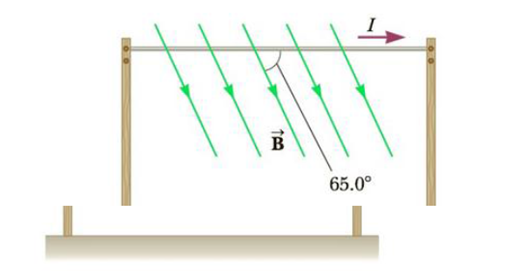Chapter 19, Problem 34P

Chapter
Section
Textbook Problem

A horizontal power line of length 58 m carries a current of 2.2 kA as shown in Figure P19.34. Earth’s magnetic field at this location has a magnitude equal to 5.0 × 10−5. T and makes an angle of 65° with the power line. Find the magnitude and direction of the magnetic force on the power line.Figure P19.34

To determine
The direction and the magnitude of the magnetic force on the power line.

Explanation

Given info: The length of the horizontal power line is 58m . The current in the power line is 2.2kA . The earth’s magnetic field has a magnitude of 5.0×105T and makes 65° with the power line at the location.

Explanation:

The magnitude of the magnetic force on a current carrying wire is given by,

Fm=BILsinθ

• B is the magnetic field
• I is the current on the wire
• L is the length of the wire
• θ is the angle between the direction of current and the magnetic field

Substitute 58m for L , 5.0×105T for B , 2.2kA for I and 65° for θ to determine the magnetic force,

Fm=(5

Still sussing out bartleby?

Check out a sample textbook solution.

See a sample solution

The Solution to Your Study Problems

Bartleby provides explanations to thousands of textbook problems written by our experts, many with advanced degrees!

Get Started

Which distance is farther, 100 km or 50 mi?

Introductory Chemistry: A Foundation

What are genomes?

Human Heredity: Principles and Issues (MindTap Course List)

What is the normal ratio of bicarbonate to carbonic acid in the blood?

Chemistry for Today: General, Organic, and Biochemistry